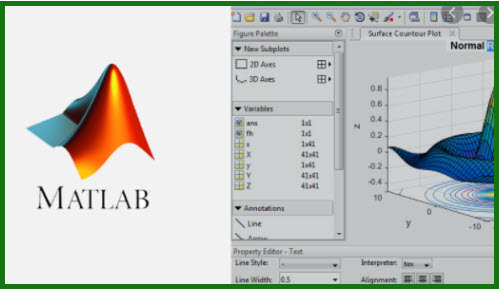Tutorials

Basic Probability applications using C++ and MATLAB

Basic Probability applications using C++ and MATLAB

Created by Dr. Spyros Giannelos . | Published 1/2021
Duration: 1 hour | 3 sections | 7 lectures | Video: 1280×720, 44 KHz | 444 MB
Genre: eLearning | Language: English + Sub

Using MATLAB and C++

What you’ll learn
This is a course that provides some fundamental coding tutorials especially for those aiming for a Quant career.
The coding practices are focused on Matlab and on C++
Understand what Stochastic Optimization is, and specifically what scenarios are and what is &quot;uncertainty&quot; and sources of uncertainty.
Understand the different levels of Uncertainty
Implement Monte Carlo Simulations
Model the histograms of different distributions

Requirements
There are no requirements. We learn, step by step, by doing.
Description
This is a course that provides some fundamental coding tutorials especially for those aiming for a Quant career.
The coding practices are focused on Matlab and on C++, both of which are important to know for applications related to Quant practices.
\n
For a deeper dive into similar topics please watch my course called “Develop Stochastic Optimization Models using GAMS&nbsp;&amp;&nbsp;FICO” .
\n
Here we focus on key probability distributions that can be used for modelling uncertainty. Also,&nbsp; we model the histograms of different distributions with the help of MATLAB. We understand what a histogram really means – this is maybe the most important diagram that you need to know how to interpret. We , therefore, look at how we can produce it and Matlab is a great tool to do so effectively.
We also quantify probabilities through Monte Carlo methods using MATLAB. Monte Carlo is a very important technique and creates much confusion, so it is important to clarify it. Therefore, we look into it as well.
In addition, using C++ we quantify probabilities by counting the number of the desired events (i.e. by using theory). Knowing how to code this in C++ will help you modify the code according to your own needs and actually go ahead and code more and more concepts.

Good luck with your career progress / career search !&nbsp;
Thank you to the hundreds of students who have enrolled to this course.